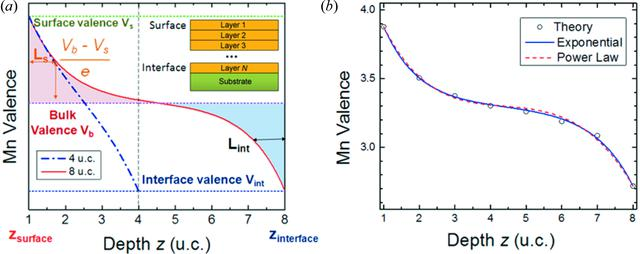disable zoom     view article Figure 6 (a) Schematic of the variation of valence with depth. Curves are shown for sample thicknesses of 4 u.c. (dash-dot line) and 8 u.c. (solid line). Inset: diagram showing layer numbers of LSMO as a function of distance from the surface for a given film thickness. Here, z = 1 is taken to be the surface Mn layer. (b) Layer-by-layer variation of valence for material thickness of 8 u.c. The data points correspond to the valence determined by theoretical calculations and the blue and red curves show the exponential and power-law fits to the data, respectively.JOURNAL OFSYNCHROTRONRADIATION
ISSN: 1600-5775
Volume 25| Part 6| November 2018| Pages 1711-1718